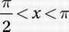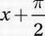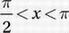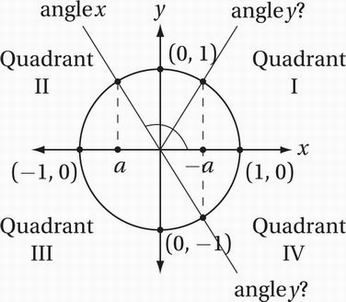# SAT Math Multiple Choice Question 688: Answer and Explanation

### Test Information

Question: 688

13. If cos x = a, where, and cos y = - a, then which of the following could be the value of y?

• A. x + 2π
• B. x + π
• C.• D. - x + 2π

Explanation:

B

Special Topics (trigonometry) HARD

The statementindicates that x is an angle in quadrant II, where the cosine is negative. Let's draw this situation on the unit circle so we can visualize it. (We don't want to confuse the angles called x and y in the problem with the x-coordinates and y-coordinates in the xy-plane. For this reason, let's label the terminal rays for the angles "angle x" and "angle y.") Recall that the cosine of any angle is the x-coordinate of the point on the unit circle that corresponds to that angle. If cos x = a, then a is the x-coordinate of the point on the unit circle that corresponds to "angle x," as shown in the diagram.Now notice that, since a is a negative number, -a (that, is, the opposite of a), is a positive number. More specifically, it is the reflection of the point labeled a over the x-axis, as shown in the diagram. Now, if cos y = -a, then "angle y" corresponds to a point on the unit circle with an x-coordinate of -a. There are two possible locations for this point on the circle, and both are shown in the diagram above. Notice that one of these angles is the reflection of "angle x" over the y-axis. This is the supplement of "angle x," that is, π - x. The other is the reflection of "angle x" over the origin, that is x + π. Therefore, the correct answer is (B).

Alternately, we could use the calculator to solve this problem by process of elimination. We can choose a value of "angle x" between π/2 and π. (In radian mode this is an angle between 1.57 and 3.14, and in degree mode it is an angle between 90° and 180°.) Let's pick "angle x" to be 2 radians (about 115°). According to the calculator, cos(2) = -.416. Therefore, cos y must equal .416. Now we can substitute x = 2 into all of the choices and see which angle has a cosine of .416.

(A) cos(2 + 2π ) = -.416

(B) cos(2 + π) = .416

(C) cos(2 + π/2) = -.909

(D) cos(-2 + 2π) = -.416

Therefore the correct answer is (B).